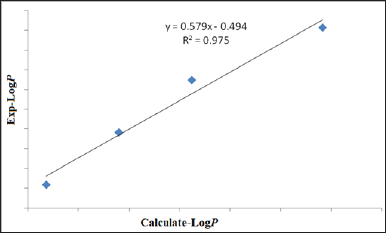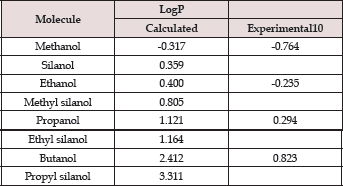info@biomedres.us   +1 (502) 904-2126   One Westbrook Corporate Center, Suite 300, Westchester, IL 60154, USA   Site Map

Impact Factor : 0.548

# Does Incorporation of Silicon Increases Lipophilicity? A Theoretical Perspective Volume 3 - Issue 1

Lakhya J Mazumder, Chayanika Kashyap, Sabnam S Ullah and Ankur K Guha*

• Department of Chemistry, Cotton University, India

Received: February 27, 2018;   Published: March 08, 2018

*Corresponding author: Ankur K Guha, Department of Chemistry, Cotton University, Guwahati, Assam, India

DOI: 10.26717/BJSTR.2018.03.000835

## Abstract

Quantum chemical calculations have been carried out using MP2/6-311++G** level of theory to calculate lipophilicity, i.e., octanol-water partition coefficient (LogP) of some alcohols and their silicon derivatives. Very good correlation has been obtained between the experimental LogP values against our calculated one. Moreover, incorporation of Si atom into the C atom attached to the -OH group dramatically increases the lipophilicity of the compounds. This finding may have wide implications towards designing new Si incorporated drugs.

## Introduction

The lipophilicity, which refers to the ability of a compound to dissolve in fats, oils, lipids and non polar solvents such as hexane or toluene, is an important parameter for a drug to successfully pass through clinical development [1-3]. The lipophilicity is a measure of all the intermolecular forces between solute and two phases between which it partitions . Generally, the lipophilicity is expressed experimentally as the octanol-water partition coefficient P (logP) or as distribution coefficients (logD). The logP describes the partition equilibrium of an un-ionized solute, while logD determines the ratio of the sum of the concentrations of all forms of the compound (pH-dependent mixture of ionized and unionized forms) in each of the two phases. Accurate determination of LogP is very important requirement in drug design. Theoretical calculation of LogP has been practiced for a long time. In practice, theoretical LogP values evaluated using quantitative structure-activity relationship (QSAR) models are practiced more often rather than expensive experimental measurements [5-6]. However, screening based on QSAR models often lead to erroneous results [7-8].

Herein, we have undertaken a theoretical study on the direct and accurate measurement of LogP values based on the following equationWhere values are the free energies in respective solvent , the calculated values of LogP were then compared with the available experimental data from Khadikar and co-workers . Recently, it has been speculated that substitution of C atom by Si increases the lipophilicity of different drugs . This is intriguing by the fact that Si is the biostere of C as Si has similar physicochemical properties and similar biological activity in broad sense. Herein, we are also interested in testing the speculation by carrying out high level abinitio calculations.

### Computational Details

All these structures were fully optimized without any symmetry constraints at MP2/6-311++G** level of theory . Harmonic frequency calculations were also carried out to understand the nature of the stationary states. All structures were found to be at the local minimum with all real frequencies. Solvent calculations were performed using the prescribed salvation model using density (SMD) approach. All calculations were performed using GAUSSIAN16 suite of program .

## Results and Discussions

Figure 1 shows the optimized geometries of the molecules considered in this study. The geometries shown here are for the gas phase. All these structures are local minima on the potential energy surface. It should be noted that many isomeric forms of these structures are possible; however, we have considered the lowest energy isomer of all these molecules. These gas phase geometries are taken as the starting geometry for further geometry optimization in different solvent (water and n-octanol) medium using SMD approach. Table 1 contains the calculated values of LogP of the selected molecules. The experimental LogP values are taken from Khadikar and co-workers . It is evident from Table 1 that the values calculated with MP2/6-311++G** level of theory lies between -0.3 to 3.3 and follows a similar trend as the experimental values. However, the magnitudes of calculated values are different from the experimental one. We, therefore, adopted regression analysis between the calculated and experimental LogP values. Figure 2 shows the plot of the regression analysis. It is evident from Figure 2 that there is a very good correlation between the calculated and experimental values with regression coefficient, R2 = 0.97. Interestingly, incorporation of Si atom into the alcohols dramatically increases the LogP value which is consistent with the recent speculation.

Figure 1: Local minimum structures of the investigated molecules calculated at MP2/6-311++G** level of theory.Figure 2: Correlation plot between the experimental and calculated LogP values.Table 1: MP2/6-311++G** calculated values of LogP.## Conclusion

Abinitio calculations at MP2/6-311++G** level of theory has been carried out for the theoretical calculation of lipophilicity parameter LogP. A nice linear correlation has been obtained for the set of alcohols studied in this work. The most important outcome of this work is the theoretical verification of the previous speculation that incorporation of Si atom into the carbon containing drugs may increase the lipophilicity and hence, may increase the physiological activity of the Si containing drugs.

## Acknowledgement

AKG thanks the Science and Engineering Research Board (SERB), Government of India for providing financial assistance in the form of a project (project no. ECR/2016/001466).